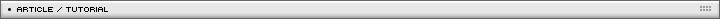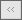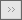Game creation tutorial Page 1/1[ February 26, 2007 ] Emanuele Feronato This is the first part of a long step-by-step article that will guide you through the entire process of creating a Flash game including input management, basic physics, collision detection and a lot more!

This is a quite long tutorial, so I decided to split it in pieces.
It started as a didactic example about flash game creation.

First of all, I got inspiration from Ball Revamped series (do not remember the link, search it on Google), but I'll add a lot more features. That means more than... two.

Let's start creating the main character.

The hero

Ok, I said I am going to create the Ball, our hero.

So at the moment our hero will be a red circle with a yellow circle inside. It looks good! Maybe you should print it and keep it on your bedroom wall.

First of all, let's think about our interaction with the hero. Basically, I want the hero to move when I press a key.

He will move to the left if I press left key, to the right if right key is pressed, and so on.

So the first hero actionscript will be:

```onClipEvent (enterFrame) {
if (Key.isDown(Key.LEFT)) {
_x--;
}
if (Key.isDown(Key.RIGHT)) {
_x++;
if (Key.isDown(Key.UP)) {
_y--;
if (Key.isDown(Key.DOWN)) {
_y++;
}
```

It's easy, isn't it?

The enterFrame event simply checks if a key is pressed, and increases or decreases the hero x or y position according to the key pressed.

Click on the movie and press arrow keys to control the hero, click on "reset" to.. hm.. reset the movie

The Power

Well, you will notice that our hero always moves at a fixed speed.

I want to set up a variable to store the speed, so if I want the hero to move faster/slower I only have to change one value.
This is very important since I am planning to develop different levels where hero speed may vary.

I need to set up a "power" variable to move the hero fastly or slowly.

```onClipEvent (load) {
power = 3;
}
onClipEvent (enterFrame) {
if (Key.isDown(Key.LEFT)) {
_x -= power;
}
if (Key.isDown(Key.RIGHT)) {
_x += power;
}
if (Key.isDown(Key.UP)) {
_y -= power;
}
if (Key.isDown(Key.DOWN)) {
_y += power;
}
}
```

Now you can change your hero's speed just adjusting the power variable.
The higher the power value, the fastest the hero movement.

The Speed

That's quite better than before, but our hero always moves at the same speed, and always starts without moving.

Now I need some kind of acceleration, and eventually some initial speed.

```onClipEvent (load) {
power = 0.2;
yspeed = 0;
xspeed = 0;
}
onClipEvent (enterFrame) {
if (Key.isDown(Key.LEFT)) {
xspeed -= power;
}
if (Key.isDown(Key.RIGHT)) {
xspeed += power;
}
if (Key.isDown(Key.UP)) {
yspeed -= power;
}
if (Key.isDown(Key.DOWN)) {
yspeed += power;
}
_y += yspeed;
_x += xspeed;
}
```

Notice how I reduced the power from the previous example (3) and this one (0.2).
Now that the power increases the speed, I need to play carefully with it or our hero will move too fast.
I have a yspeed and xspeed values starting at 0, but I can change them to make the hero move as he enters the frame.

Now, the more you press a key, the more your hero gains speed, the harder is to change direction.

Good.

The Friction

But now I want a friction, because I do not want the hero to move forever if I don't press any key.
I want the hero to slowly stop moving if no key is pressed.

```onClipEvent (load) {
power = 0.3;
yspeed = 0;
xspeed = 0;
friction = 0.95;
}
onClipEvent (enterFrame) {
if (Key.isDown(Key.LEFT)) {
xspeed -= power;
}
if (Key.isDown(Key.RIGHT)) {
xspeed += power;
}
if (Key.isDown(Key.UP)) {
yspeed -= power;
}
if (Key.isDown(Key.DOWN)) {
yspeed += power;
}
xspeed *= friction;
yspeed *= friction;
_y += yspeed;
_x += xspeed;
}
```

So I introduced a friction, minor than 1, that will affect hero's speed. Now, when I press a key the hero starts moving, but when I release the key the hero will slowly stop. How slowly? The higher the friction (always minor than 1, remember), the slowly our hero will decrease his speed.

The Gravity

I said I wanted to do something similar to Ball Revamped, so I need a gravity. The hero will fall according to gravity strenght.

``` onClipEvent (load) {
power = 0.3;
yspeed = 0;
xspeed = 0;
friction = 0.95;
gravity = 0.1
}

onClipEvent (enterFrame) {
if (Key.isDown(Key.LEFT)) {
xspeed -= power;
}
if (Key.isDown(Key.RIGHT)) {
xspeed += power;
}
if (Key.isDown(Key.UP)) {
yspeed -= power;
}
if (Key.isDown(Key.DOWN)) {
yspeed += power;
}
xspeed *= friction;
yspeed += gravity;
_y += yspeed;
_x += xspeed;
}
```

So I replaced the friction with the gravity for vertical movements. If I do not press UP arrow, the hero will fall down. When you read this line, probably the hero has fallen outside of the movie (you'll see how to prevent this later in the tutorial), so you may need to click on reset to place the hero at the center of the movie.

need to click on reset to place the hero at the center of the movie.

The Thrust

If I have gravity, I need a thrust. I want to be more complicated to move up since my hero is living in a world where gravity exists.

``` onClipEvent (load) {
power = 0.3;
yspeed = 0;
xspeed = 0;
friction = 0.95;
gravity = 0.1;
thrust = 0.75;
}

onClipEvent (enterFrame) {
if (Key.isDown(Key.LEFT)) {
xspeed -= power;
}
if (Key.isDown(Key.RIGHT)) {
xspeed += power;
}
if (Key.isDown(Key.UP)) {
yspeed -= power*thrust;
}
if (Key.isDown(Key.DOWN)) {
yspeed += power*thrust;
}
xspeed *= friction;
yspeed += gravity;
_y += yspeed;
_x += xspeed;
}
```

As you can see, thrust (again minor than 1) affects the UP movement, making it weaker and more complicated to accomplish

The Wind

What's now? Sure, the wind!
I want to make the stage look windy, you know, heros' life is complicate, so that's it!

``` onClipEvent (load) {
power = 0.3;
yspeed = 0;
xspeed = 0;
friction = 0.95;
gravity = 0.1;
thrust = 0.75;
wind = 0.09;
}
onClipEvent (enterFrame) {
if (Key.isDown(Key.LEFT)) {
xspeed -= power;
}
if (Key.isDown(Key.RIGHT)) {
xspeed += power;
}
if (Key.isDown(Key.UP)) {
yspeed -= power*thrust;
}
if (Key.isDown(Key.DOWN)) {
yspeed += power*thrust;
}
xspeed += wind;
xspeed *= friction;
yspeed += gravity;
_y += yspeed;
_x += xspeed;
}
```

Wind affects side movements, if positive will move the hero to the right, if negative will move the hero to the left.
Play with all those variables wisely and find a perfect mix of realism and playability.

The Rotation

Now, the final touch. Just like in Ball Revamped, I want the hero to rotate clockwise when moving to right, and counter clockwise when moving to left.

``` onClipEvent (load) {
power = 0.65;
yspeed = 0;
xspeed = 0;
friction = 0.99;
gravity = 0.1;
thrust = 0.75;
wind = 0.05;
}
onClipEvent (enterFrame) {
if (Key.isDown(Key.LEFT)) {
xspeed -= power;
}
if (Key.isDown(Key.RIGHT)) {
xspeed += power;
}
if (Key.isDown(Key.UP)) {
yspeed -= power*thrust;
}
if (Key.isDown(Key.DOWN)) {
yspeed += power*thrust;
}
xspeed += wind;
xspeed *= friction;
yspeed += gravity;
_y += yspeed;
_x += xspeed;
_rotation += xspeed;
}
```

That's easy, I only need to se the hero rotation equal to his xspeed.

This is where the first part ends... leave me feedback and tell me what do you think about it.

Here it is a zipped file with all source codes explained in this tutorial.Name: Emanuele Feronato Location: Venice, Italy Age: 35 Flash experience: 2 years Job: Web developer and programming teacher Website: http://www.emanueleferonato.com| Homepage | News | Games | Articles | Multiplayer Central | Reviews | Spotlight | Forums | Info | Links | Contact us | Advertise | Credits || www.smartfoxserver.com | www.gotoandplay.biz | www.openspace-engine.com | gotoAndPlay() v 3.0.0 -- (c)2003-2008 gotoAndPlay() Team -- P.IVA 03121770048